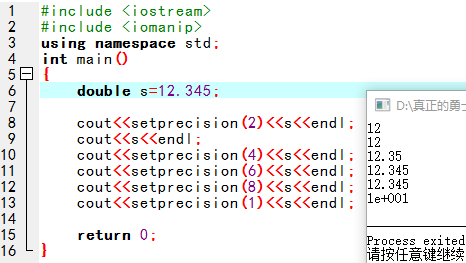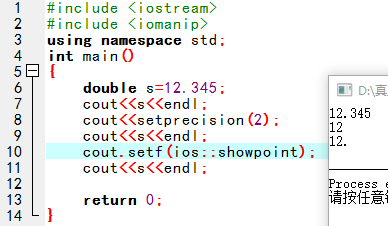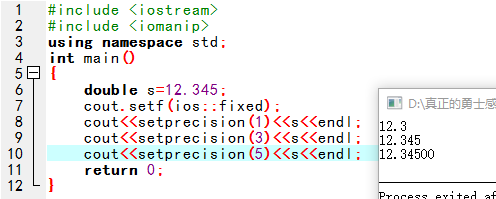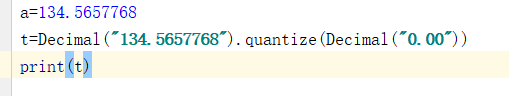• 做题遇到保留两位小数的题目，课本上写的又多又杂，网上查来的也是一堆内容需要筛选，눈_눈还是自己总结一下吧。 　首先说C++代码 #include &amp;amp;amp;amp;lt;iomanip&amp;amp;amp;amp;gt...
做题遇到保留两位小数的题目，课本上写的又多又杂，网上查来的也是一堆内容需要筛选，눈_눈还是自己总结一下吧。
首先说C++代码
	#include <iomanip>  //不要忘了头文件

	//第一种写法
cout<<setiosflags(ios::fixed)<<setprecision(2);

	//第二种写法
cout.setf(ios::fixed);
cout<<setprecision(2);

	//第三种写法
cout<<fixed<<setprecision(2);

要保留几位小数setprecision(n)的括号里n就换成几。
前两种写法是一样的，第三种是简化写的。
上面的语句写一次就行了，对之后的数字都有效。
然后说C的代码
个人感觉c的输出比较简单粗暴。
%f 格式化输出浮点型数据，在%之后加上“.n”即可。例如：
#include  <stdio.h>
int main()
{
float PI=3.1415926;
float R=5.3;
printf("面积 = %.2f\n", PI * R * R); //输出：面积 = 88.25
printf("面积 = %f\n", PI * R * R);   //输出：面积 = 88.247337
printf("面积 = %.8f\n", PI * R * R); //输出：面积 = 88.24733734
return 0;
}

%.2f\n中的“.2”即保留两位小数
//不设定保留几位小数，则默认六位
如果单纯会用就行，看到这里就可以了！
想深入理解的，继续看下面눈_눈
1.首先解释一下“语句写一次就行了，对之后的数字都有效”。在s之后设置保留两位小数之后，重新声明另一个数，输出依旧显示两位小数。所以设置精度语句只需写一次就可以了。
#include <iostream>
#include <iomanip>　　　//设置必备的头文件
using namespace std;
int main()
{
double s=12.345;
cout<<setiosflags(ios::fixed)<<setprecision(2);
cout<<s<<endl;　　　//输出12.35

float pi=3.14159;
cout<<pi<<endl;　　　//输出3.14

return 0;
}

2.setprecision(n)
功能：控制浮点数显示的有效数字个数。
图中可以看出，只用setprecision(n)是控制保留几位有效数字的。
由8-9两行代码可以看出，也是只写一次就可以。
8-10行可以看出，只是四舍五入修改了数字的显示方法，并不是修改原数字。从常识我们可以知道，如果12.345数字本身改变，那就是两位有效数字变为 12，那从两位有效数字改为四位有效数字会变为 12.00，而不是12.34。
11-12行可以看出如果要保留的太多，是不会补上0的（往下看有补0的方法）。
13行中可以看出，如果小数点前的位数多于你要保留位数，则会使用科学计数法。2.5补充一小点showpoint这是在尝试过程中发现的一个无聊操作，如果12.34保留两位有效数字，会显示12，但是你可以经过这个操作让它显示12. 哈哈哈哈哈哈哈注意有个“.”ヽ(•̀ω•́ )ゝ！
	double s=12.345;
cout<<s<<endl;　　　//输出原数12.345
cout<<setprecision(2);
cout<<s<<endl;　　　//输出保留两位有效数字12
cout.setf(ios::showpoint);//或者写cout<<setiosflags(ios::showpoint);
cout<<s<<endl;　　　//输出保留两位有效数字外加一个小数点12.

3.fixed
setprecision(n)和fixed合用的话可以控制小数点后有几位。
只要加上以下任意一个语句就可以。
cout<<setiosflags(ios::fixed);
cout.setf(ios::fixed);
cout<<fixed;

然后你会发现，如果你要保留的位数多于数字原来的小数，就会补上0。_(:3 」∠) 我是萝莉安，梦想是做个程序媛……
有CSDN账号的帮我点个赞或者评论一下吧，不愿意也不强求啦。
你们点赞评论我才会得积分，才会升等级，等级高了才能给博客换好看的皮肤🥰🥰


展开全文• java保留两位小数5种方法 四舍五入，例： import java.math.BigDecimal; import java.text.DecimalFormat; import java.text.NumberFormat; public class format { double f = 111231.5585; public void m1() {...
java保留两位小数5种方法 四舍五入，例：
import java.math.BigDecimal;
import java.text.DecimalFormat;
import java.text.NumberFormat;

public class format {
double f = 111231.5585;

/**
* BigDecimal转double 四舍五入保留两位小数
*/
public void m1() {
BigDecimal bg = new BigDecimal(f);
double f1 = bg.setScale(2, BigDecimal.ROUND_HALF_UP).doubleValue();
System.out.println(f1);
}
/**
* BigDecimal除法 四舍五入保留四位小数
*/
public void m2() {
BigDecimal number = new BigDecimal(smsIncome).divide(new BigDecimal(smsIncomeChina), 4, RoundingMode.HALF_UP)
}
/**
* DecimalFormat转换最简便
*/
public void m3() {
DecimalFormat df = new DecimalFormat("#.00");
System.out.println(df.format(f));
}
/**
* String.format打印最简便
*/
public void m4() {
System.out.println(String.format("%.2f", f));
}
public void m5() {
NumberFormat nf = NumberFormat.getNumberInstance();
nf.setMaximumFractionDigits(2);
System.out.println(nf.format(f));
}

public static void main(String[] args) {
format f = new format();
f.m1();
f.m2();
f.m3();
f.m4();
f.m5();
}
}



展开全文• round(a,2)=12.35 保留两位小数 round(a,2)=12.35 保留三位小数 方法2， 例如： a=12.34567889 print（“%.2f”%a) 保留两位小数 print（“%.3f”%a) 保留三位小数 print（“%.4f”%a) 保留四位小数 方法3， 首先...
方法1，用round函数
例如:
a=12.34567889
round(a,2)=12.35    保留两位小数
round(a,2)=12.35    保留三位小数
方法2，
例如：
a=12.34567889
print（“%.2f”%a)  保留两位小数
print（“%.3f”%a)  保留三位小数
print（“%.4f”%a)  保留四位小数
方法3，
首先引入decima函数
from decimal import Decimal
a=134.5657768
t=Decimal(“134.5657768”).quantize(Decimal(“0.00”))
print(t)输出结果：
134.57


展开全文• js保留两位小数的函数有：1、【toFixed()】函数；2、【Math.floor()】函数不四舍五入 ，向下取整；3、使用字符串匹配方法；4、四舍五入保留2位小数；5、浮点数保留两位小数。js保留两位小数的函数有：一、四舍五入...
js保留两位小数的函数有：1、【toFixed()】函数；2、【Math.floor()】函数不四舍五入 ，向下取整；3、使用字符串匹配方法；4、四舍五入保留2位小数；5、浮点数保留两位小数。js保留两位小数的函数有：一、四舍五入相关1、toFixed()方法需注意，保留两位小数，将数值类型的数据改变成了字符串类型// 1.四舍五入var num =2.446242342;num = num.toFixed(2);console.log(num); //2.45console.log(typeof num); // string2、Math.floor()，不四舍五入 ，向下取整注意，不改变数据类型// 2.不四舍五入 向下取整num = Math.floor(num * 100) / 100;console.log(num); //2.44console.log(typeof num); // number3、字符串匹配注意，先将数据转换为字符串，最后再转为数值类型// 3.不四舍五入 字符串匹配再转换num = Number(num.toString().match(/^\d+(?:\.\d{0,2})?/));console.log(num); //2.44console.log(typeof num); // number4、四舍五入保留2位小数(若第二位小数为0，则保留一位小数)注意，数据类型不变//4.四舍五入保留2位小数(若第二位小数为0，则保留一位小数)function keepTwoDecimal(num) {var result = parseFloat(num);if (isNaN(result)) {alert('传递参数错误，请检查！');return false;}result = Math.round(num * 100) / 100;return result;};keepTwoDecimal(num);console.log(num); //2.44console.log(typeof num); //number5、四舍五入保留2位小数(不够位数，则用0替补)注意，数据类型变为字符串类型//5.四舍五入保留2位小数(不够位数，则用0替补)function keepTwoDecimalFull(num) {var result = parseFloat(num);if (isNaN(result)) {alert('传递参数错误，请检查！');return false;}result = Math.round(num * 100) / 100;var s_x = result.toString(); //将数字转换为字符串var pos_decimal = s_x.indexOf('.'); //小数点的索引值// 当整数时，pos_decimal=-1 自动补0if (pos_decimal < 0) {pos_decimal = s_x.length;s_x += '.';}// 当数字的长度< 小数点索引+2时，补0while (s_x.length <= pos_decimal + 2) {s_x += '0';}return s_x;}console.log(keepTwoDecimalFull(120.5)); //120.50console.log(typeof keepTwoDecimalFull(120.5)); //stringconsole.log(keepTwoDecimalFull(2.446242342)); //2.45console.log(typeof keepTwoDecimalFull(2.446242342)); //string二、浮点数保留两位小数1、将浮点数四舍五入，取小数点后2位注意，数据类型不变//浮点数保留两位小数//1.功能：将浮点数四舍五入，取小数点后2位function toDecimal(x) {var f = parseFloat(x);if (isNaN(f)) {return;}f = Math.round(x*100)/100;return f;}console.log(toDecimal(3.1465926)); // 3.15console.log(typeof toDecimal(3.1415926)); //number2、强制保留2位小数，如：2，会在2后面补上00.即2.00注意，数据类型变为字符串类型//2.强制保留2位小数，如：2，会在2后面补上00.即2.00function toDecimal2(x) {var f = parseFloat(x);if (isNaN(f)) {return false;}var f = Math.round(x*100)/100;var s = f.toString();var rs = s.indexOf('.');if (rs < 0) {rs = s.length;s += '.';}while (s.length <= rs + 2) {s += '0';}return s;}console.log(toDecimal2(3.1)); // 3.10console.log(typeof toDecimal2(3.1415926)); //string3、保留两位小数 浮点数四舍五入 位数不够 不补0注意，数据类型不变// 3.保留两位小数 浮点数四舍五入 位数不够 不补0function fomatFloat(src,pos){return Math.round(src*Math.pow(10, pos))/Math.pow(10, pos);}console.log(fomatFloat(3.12645,2)); // 3.13console.log(typeof fomatFloat(3.1415926)); //numbe更多相关免费学习推荐：javascript视频教程
展开全文• php转化为两位小数的方法：1、利用“round()”对浮点数进行四舍五入；2、利用“sprintf”格式化字符串；3、利用千位分组来格式化数字的函数“number_format()”。推荐：《PHP视频教程》PHP保留两位小数的三种方法/**...
• Java中，当两个整数相除时，由于小数点以后的数字会被截断，运算结果将为整数，此时若希望得到运算结果为浮点数，必须将两整数其一或是两者都强制转换为浮点数，也就是Java输出怎么保留两位小数?接下来郑州达内Java...
• ## Python中保留两位小数的几种方法

万次阅读 多人点赞 2018-12-14 21:43:57
保留两位小数，并做四舍五入处理 方法一: 使用字符串格式化 &gt;&gt;&gt; a = 12.345 &gt;&gt;&gt; print("%.2f" % a) 12.35 &gt;&gt;&gt; 方法二: 使用round内置...round Decimal python 字符串格式化
• js限制输入数字能输入小数点，js定义数组，js往数组中添加数据，js将字符型转为数字型，除法结果保留两位小数——js小测：计算比赛得分一个朋友跟我说要去给某个比赛算分: 规则:去掉最低分最高分求平均分: 最近在...
• ## Sql 保留两位小数

万次阅读 2019-05-16 17:51:25
使用 Round() 函数，如 Round(number,2) ，其中参数2表示保留两位有效数字，但是只负责四舍五入到两位小数，但是不负责截断 例如 ROUND(3.141591653, 2) 得到3.140000000； 使用 Convert(decimal(10,2),number) ...
• 以形助数，为数代言——关于《小数乘小数》的...这些素材从生活中提取，一位小数乘一位小数、两位小数乘一位小数蕴含在一道题中，有助于学生细分问题、有序思考、分步解决，但在实际教学中，学生往往将小数点的位置...
• Java使double保留两位小数的多方法 Java保留两位小数 方式一：保留两位小数 DecimalFormat df = new DecimalFormat("#.00"); double d1 = 1.23456 double d2 = 2.0; double d3 = 0.0; ...
• 这里写自定义目录标题double保留两位小数,如果小数最后一位是0,则保留一位小数 double保留两位小数,如果小数最后一位是0,则保留一位小数 /** * double转换 */ private String doubleTrans(double d) { if (Math...
• el表达式保留两位小数、使用jstl fmt formatNumber标签保留两位小数
• 这里有三种方法，round(a,2)'%.2f' % aDecimal('5.000').quantize(Decimal('0.00'))当需要输出的结果要求有两位小数的时候，字符串形式的：'%.2f' % a 方式最好，其次用Decimal。需要注意的：1. 可以传递给Decimal...
• 本文是小编针对js保留两位小数这个大家经常遇到的经典问题整理了在各种情况下的函数写法以及遇到问题的分析，以下是全部内容：一、我们首先从经典的“四舍五入”算法讲起1、四舍五入的情况var num =2.446242342;...
• Python中实现保留两位小数的方法如下：保留两位小数，并做四舍五入处理方法一: 使用字符串格式化>>> a = 12.345>>> print("%.2f" % a)12.35>>>方法二: 使用round内置函数>>> a ...
• ## Java中保留两位小数

万次阅读 2019-09-09 19:53:05
保留两位小数 //s2是要保留两位小数的数，%.2f 指的是保留两位小数 double s1 = String.format("%.2f",s2)
• python输入保存两位小数的四种办法：a = 5.5461办法一：round(a,2)办法二：float('%.2f' % a)相干保举：《Python视频教程》办法三：‘%.2’ %a办法四：from decimal import DecimalDecimal('5.026').quantize...
• VC++浮点数四舍五入保留两位小数VC++浮点数四舍五入保留两位小数
• 原spring boot 对double保留两位小数一：全局设置。package com.mszl.utils;import com.fasterxml.jackson.core.JsonGenerator;import com.fasterxml.jackson.databind.JsonSerializer;import ...
• 这里有三种方法，round(a,2)'%.2f' % aDecimal('5.000').quantize(Decimal('0.00'))当需要输出的结果要求有两位小数的时候，字符串形式的：'%.2f' % a 方式最好，其次用Decimal。需要注意的：1. 可以传递给Decimal...
• 项目中遇到小数相似度（业务给过来是小数）需要web界面以百分数的形式表示，并且保留两位小数。 直接上代码。 var nval = 0.12345; var percent = (Math.round(nval*10000))/100+'%'; 说明： 1.Math.round(num)...javascript
• Android输入框限制两位小数，先来看看效果图 直接上代码，没有太多的神奇就是监听了输入框EditText的输入监听事件而已 picET.addTextChangedListener(new TextWatcher() { @Override public void ...
• 对于金额的显示，大多情况下需要保留两位小数，比如下面的(表格采用 element-ui)：在vue.js中，对文本的处理通常是通过设置一系列的过滤器，过滤器可以用在两个地方：双花括号插值 和 v-bind 表达式 (后者从 2.1.0+ ...
• Vue toFixed保留两位小数的3种方式第一种：直接写在js里面，这是最简单的val.toFixed(2)第二种：在ElementUi表格中使用第三种：在取值符号中使用 {{}}定义一个方法towNumber(val) {return val.toFixed(2)}使用{{ ...
• 今天被zz问到怎么去保留两位小数，心里默默吐槽下，搞什么，自己去查Math的API好吗…哼…. 为了进一步证明他的zz，我就去查API，发现……额……，Math这个类真的没有保留两位小数的方法!!! 唔，看来比较zz的是我和...
• ## Lua中保留两位小数

千次阅读 2020-04-18 11:41:46
在使用Lua进行开发的时候，经常会遇到保留n位小数的问题，这里以保留两位小数为例，记录一下需要注意的地方！ 在选择处理方案之前，首先要确认需求，搞清楚保留两位小数是指小数点后第三位四舍五入还是从小数点后第...lua
• 在写程序的时候，有时候可能...本文以两位小数为例给出四种方法。package CodeJava_Leet;import java.math.BigDecimal;import java.text.DecimalFormat;import java.text.NumberFormat;/*** Created by Yechengpeng ...
• ## java 两个整数相除保留两位小数

万次阅读 多人点赞 2016-11-28 11:15:32...